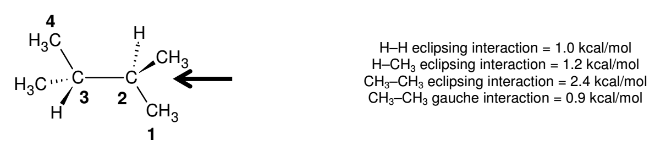# Problem: What is the total strain energy (in kcal/mol) of the least stable (highest energy) conformation possible for 2,3-dimethylbutane? All of the choices below are expressed in units of kcal/mol.   A. 0          B. 0.9          C. 1.8           D. 2.7         E. 3.4 F. 3.6        G. 4.4         H. 5.8           I. 7.2           J. None of these

###### FREE Expert Solution
94% (281 ratings)
###### Problem Details

What is the total strain energy (in kcal/mol) of the least stable (highest energy) conformation possible for 2,3-dimethylbutane? All of the choices below are expressed in units of kcal/mol.

A. 0          B. 0.9          C. 1.8           D. 2.7         E. 3.4

F. 3.6        G. 4.4         H. 5.8           I. 7.2           J. None of these